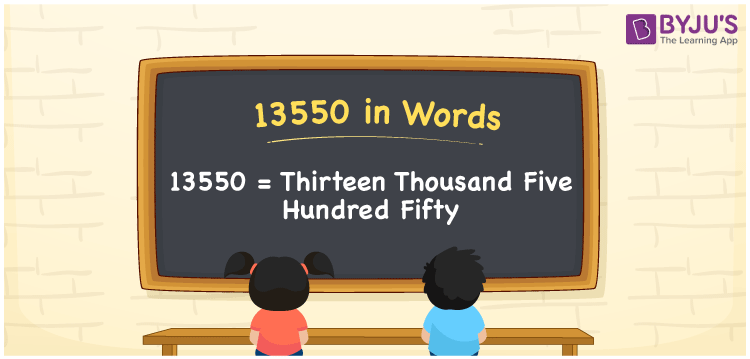# 13550 in Words

13550 in words is written as Thirteen thousand five hundred fifty. In both the International System of Numerals and the Indian System of Numerals, 13550 is written as Thirteen thousand five hundred fifty. The number 13550 is a Cardinal Number as it represents some quantity. For example, “monthly rent of this place is 13550 rupees”.

 13550 in Words Thirteen thousand five hundred fifty Thirteen thousand five hundred fifty in Number 13550

## 13550 in English Words

13550 in English words is read as “Thirteen thousand five hundred fifty”.## How to Write 13550 in Words?

To write 13550 in words, we shall use the place value chart. In the place value chart, put 1 in the ten thousand, 3 in the thousands, 5 in the hundreds and tens, and 0 in the ones. Let us make a place value chart to write the number 13550 in words.

 Ten Thousands Thousands Hundreds Tens twos 1 3 5 5 0

Thus, we can write the expanded form as

1 × Ten Thousand + 3 × Thousand + 5 × Hundred + 5 × Ten + 0 × One

= 1 × 10000 + 3 × 1000 + 5 × 100 + 5 × 10 + 0 × 1

= 10000 + 3000 + 500 +50 + 0

= 13550

= Thirteen thousand five hundred fifty.

13550 is a natural number, the successor of 13549 and the predecessor of 13551.

13550 in words – Thirteen thousand five hundred fifty

• Is 13550 an odd number? – No
• Is 13550 an even number? – Yes
• Is 13550 a perfect square number? – No
• Is 13550 a perfect cube number? – No
• Is 13550 a prime number? – No
• Is 13550 a composite number? – Yes

## Frequently Asked Questions on 13550 in Words

Q1

### How to write 13550 in words?

13550 in words is written as Thirteen thousand five hundred fifty.
Q2

### How to write 13550 in the International and Indian System of Numerals?

In both, the system of numerals, 13550 in words, is written as Thirteen thousand five hundred fifty.
Q3

### How to write 13550 in a place value chart?

In the place value chart, write 1 in the ten thousand, 3 in the thousands, 5 in the hundreds and tens, and 0 in the ones.